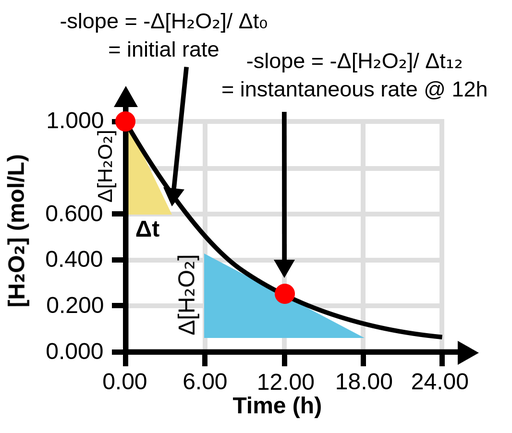The reaction rate is not constant, but changes over time. This is because the concentration changes, and the concentration affects the reaction rate.Figure 1. The reaction rate can be determined by the change of the H2O2 concentration over time. The instantaneous rate at 12 h can be obtained by computing the slope of a line tangent to the curve at that time. The initial rate is the instantaneous rate at t = 0.

• The average rate of reaction: The change in concentration of product or reactant divided over the time interval considered.

• The instantaneous rate: The reaction rate at a given time point.

• The initial rate: The instantaneous rate of a reaction at “time zero”, that is, at the beginning of the reaction.